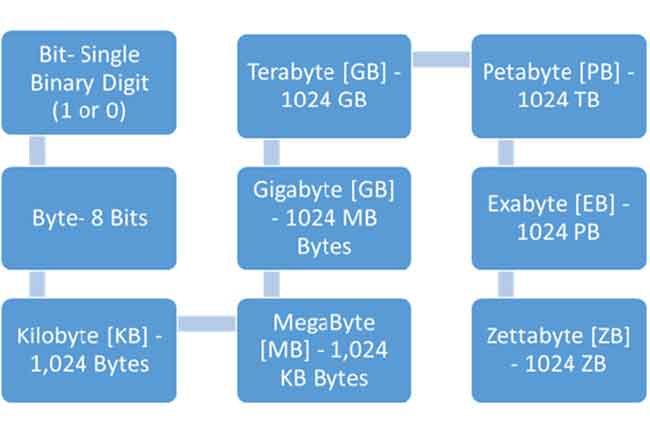The units Mbps,Kbps,bps,kb,mb are often mentioned in CCTV network surveillance. Do you know what's Mbps,Kbps,bps,kb,mb stand for?Here we'd like to explain about them.Mbps: Million bit per second

Kbps: Kilo bit per second

bps: bit per second

bit is speed unit,usually is expressed in b(lower case),refers to single binary digit(1 or 0).

Million bit = 1000 Kilobit = 1000000 bit, 1Mbps = 1000 000bps

This unit is typically used to measure bandwidth, it refers to the number of bits transmitted per second;

Usually,the rate displays on software refers to the number of Bytes transmitted per second expressed in B (capital);

MB:Million Bytes

KB:Kilobytes

B:Byte

1MB = 1024KB = 1000*1024B;

1B=8b

1M bandwidth refers: 1Mbps = 1000Kbps = 1000/8KBps = 125KBps；

So download speed of 1M bandwidth does not exceed 125KB per second.

2M bandwidth is 250KBps,3MP bandwidth is 375KBps.